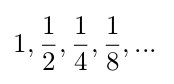# Recursion: basic-level questions

If you need help reviewing Recursion, take a look at these resources:

Each question has a "Toggle Solution" button -- click it to reveal that question's solution.

## Conceptual Questions

### Question 1

What is a recursive function?

A recursive function is a function that calls itself one or more times. The call to itself is called a recursive call.

### Question 2

Explain what base cases and recursive cases are. How do they interact with each other?

A base case is an input that requires no recursive calls to compute; the output for that input is "immediately" known. A recursive case is an input that requires one or more recursive calls to compute an output. Since each recursive case makes a call to the function again, it eventually needs a stopping point: that stopping point is the base case."""

### Question 3

What is a tree recursive function? How is it different from a linearly recursive function?

A tree recursive function is a function that makes two more recursive calls to itself (for example, the `fibonacci` function). This is different from linearly recursive functions (such as `factorial`), because linearly recursive functions make exactly one recursive call.

### Question 4

Describe what is wrong with the following function and find a way to fix it:

``````def factorial(n):
return n * factorial(n)``````

There are two problems with `factorial`.

1. First, the recursive call is made on the exact same input `n`. Since the input never gets smaller, the function will loop forever. To fix it, change the argument to the recursive call from `n` to `n-1`.
2. There is no base case. There's no stopping point, so it will run forever. To fix it, add a base case for `n == 0`

Notice that you need to fix both of these problems for it to work, otherwise the function will still loop forever!

``````def factorial(n):
if n == 0:
return 1
else:
return n * factorial(n - 1)``````

## Code-Writing questions

### Question 5

A geometric series is a series whose first element is some number a; each element is equal to the previous element multiplied by some other number r. For example, the seriesis a geometric series with the first element a = 1 and ratio r = 1/2.

Implement a function `geo_sum` that takes three numbers `a` and `r` (as defined above) and `n`, and calculates the sum of the first `n` elements of the geometric series defined by `a` and `r`. Use recursion!

``````def geo_sum(a, r, n):
"""Returns the first n elements of a geometric series.

>>> geo_sum(1, 1/2, 4)  # 1 + 1/2 + 1/4 + 1/8
1.875
>>> geo_sum(2, 2, 3)  # 2 + 4 + 8
14
"""
``````def geo_sum(a, r, n):
if n == 1:
return a
else:
return a + geo_sum(a*r, r, n-1)``````

### Question 6

Implement a function `num_primes` which takes a number `n` and returns the number of prime numbers less than or equal to `n`. You can assume there is already a function `is_prime` that takes in a number i and returns `True` if i is prime, and `False` otherwise. Use recursion!

``````def num_primes(n):
\"\"\"Returns the number of primes less than or equal to n.

>>> num_primes(6)   # 2, 3, 5
3
>>> num_primes(13)  # 2, 3, 5, 7, 11, 13
6
\"\"\"

def is_prime(i):
m = 2
while m * m <= i:
if i % m == 0:
return False
m += 1
return True``````
``````def num_primes(n):
if n < 2:
return 0
elif is_prime(n):
return 1 + num_primes(n - 1)
else:
return num_primes(n - 1)``````

### Question 7

Implement a function `any` which takes two numbers `a` and `b` and a predicate function `pred`, and returns `True` if any number from `a` to `b` inclusive satisfies `pred` (i.e. return `True` if there exists some number i such that `a < i < b` and `pred(i)` returns `True`). You may assume `a < b`. Use recursion!

``````def any(a, b, pred):
"""Returns True if any numbers from a to b inclusive satisfy
pred.

>>> any(1, 4, lambda x: x % 2 == 0)
True
>>> any(-5, 2, lambda x: x * x == -3 * x)   # -3 satisfies pred
True
>>> any(1, 6, lambda x: x % 7 == 0)
False
>>> any(0, 6, lambda x: x % 7 == 0)
True
"""
``````def any(a, b, pred):